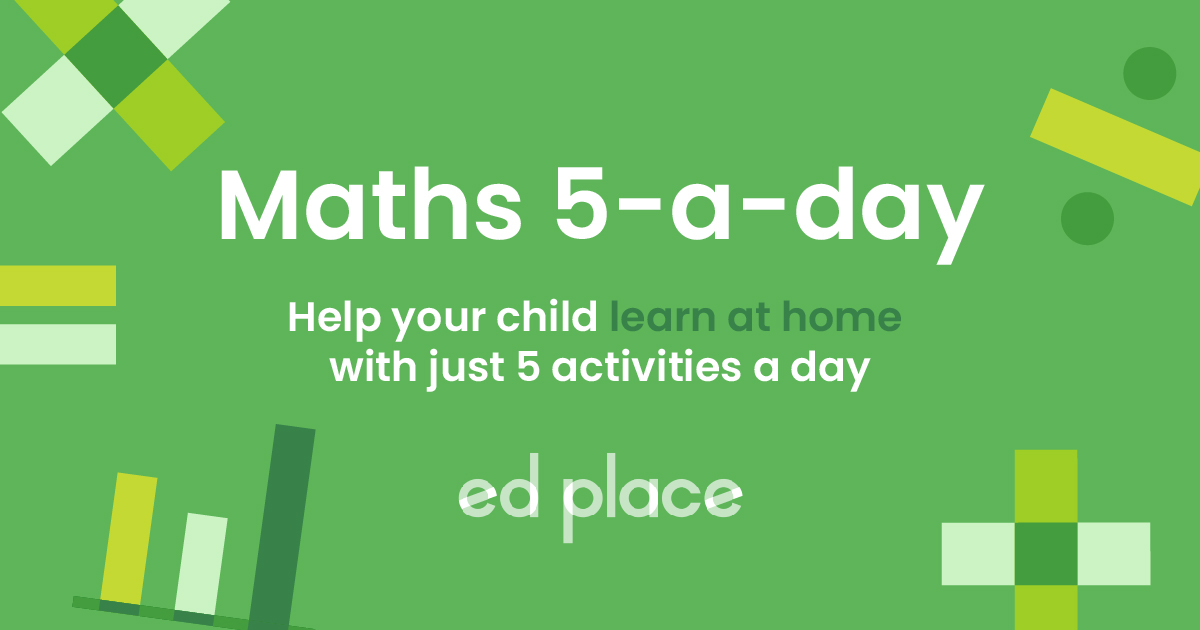# EdPlace's Year 2 Home Learning Maths Lesson: Factors

Looking for short lessons to keep your child engaged and learning? Our experienced team of teachers have created English, maths and science lessons for the home, so your child can learn no matter where they are.  And, as all activities are self-marked, you really can encourage your child to be an independent learner. Get them started on the lesson below and then jump into our teacher-created activities to practice what they've learnt. We've recommended five to ensure they feel secure in their knowledge - 5-a-day helps keeps the learning loss at bay (or so we think!).

Are they keen to start practising straight away? Head to the bottom of the page to find the activities.

Now...onto the lesson!

Key Stage 2 Statutory Requirements for Maths
Year 2 students should be able to recognise and use factor pairs.

# Is your child confused by factors and how they link to multiplication and division?

Factors. What are they used for and why does your child need to know them? As adults, we often use factors in everyday life without realising yet they’re a common area of confusion for children who struggle with mental division and multiplication.

At EdPlace we’re surrounded by a team of experts who communicate these concepts with children on a day-to-day basis, and we’re ready to share their teaching gems with you.  Follow the step-by-step approach below to make working with factors as easy as pie!

1) Understand what factors are

2) Apply this understanding to find factor pairs of a given 2-digit number

3) Explain how to use a systematic approach to ensure they don't miss out any factors.

## Step 1 - Key terminology

Before we start finding factors it’s important to check that your child understands what the key terminology means.

A factor is a number which fits exactly into another number without any remainders. Factors usually come in pairs so most numbers have an even number of factors. However, squared numbers will have an odd number of factors because they can be made by multiplying a number by itself.

For example, the number 6 would have 4 factors: 1 and 6, 2 and 3.

We can multiply 1 × 6 and 2 × 6 to get 6. So, it has an even number of factors.

The number 9 would have 3 factors: 1 and 9, and 3.

We can multiply 1 × 9 and 3 × 3 to get 9. This means it has an odd number of factors.

## Step 2 - What do we mean by division?

When working with factors it is essential that your child has a good, solid understanding of what we mean by dividing. A really easy way of explaining this to your child is to practise sharing out objects into equal sized groups. For example, I have 12 sweets, how can we share them equally? If we share them into 2 groups, how many will be in each group?

In year 3, your child will have learned their 3, 4 and 8 times tables and should also already know their 2, 5 and 10 times tables. They will also have been taught to recognise and recall the related division facts e.g. 3 × 4 = 12 and 4 × 3 = 12 so we also know that 12 ÷ 3 = 4 and 12 ÷ 4 = 3.

To be able to find factors of a number, it’s really important that children have a good recall of their multiplication and related division facts. They will also need to understand the concept of sharing into equal groups for division and repeated addition for multiplication.

## Step 3 - The systematic approach (it's not as scary as it sounds!)

When finding factors of a number, we can use a systematic approach to ensure we don’t miss any out. One way of doing this is to work in numerical order to find multiplications with the same total. These will give us factors!

To do this, we need to find factor pairs. These are pairs of numbers which can be multiplied together to make the number.

To find factors of 24, start with the number 1 and keep finding factor pairs until you reach half of 24 (12).## Step 4 - Putting it into practise...

Why not apply the method above to the following factor questions together?

1) Find the factors of the number 20.

2) Which factors of 40 are missing from this list? 2, 4, 5, 8, 10, 20

3) Beth says that the number 30 has 10 factors. Is she correct? Can you explain why?

4) David makes a list of numbers 0-100 which have an odd number of factors. He has included 100, 64 and 16 so far. Which other numbers could he include?

## Step 5 - Give it a go...

Now that you’ve covered this lesson together, why not put this to the test and assign your child the following factor-related activities in this order? All activities are created by teachers and automatically marked. Plus, with an EdPlace subscription, we can automatically progress your child at a level that's right for them. Sending you progress reports along the way so you can track and measure progress, together - brilliant!

1) 20 has the factors 1, 2, 4, 5, 10 and 20 (1 x 20, 2 x 10, 4 x 5).

2) The numbers 1 and 40 are missing. It’s important to remember that all numbers have themselves as a factor, plus the number 1!

3) Beth is not correct. The number 30 has eight factors: 1, 2, 3, 5, 6, 10, 15, 30.

4) David could include 1, 4, 9, 25, 36, 49, and 81. These are all square numbers, so will have an odd number of factors as one-factor pair is a number multiplied by itself e.g. 3 x 3 = 9.

Keep going! Looking for more activities, different subjects or year groups?

Click the button below to view the EdPlace English, maths, science and 11+ activity library